Next: 2.2 Experimental setup for Up: 2.1 The basics Previous: 2.1 The basics

### Detection of positrons

To reconstruct the muon spin direction, it is convenient to have two positron counters which are placed symmetrically with respect to the sample (muon) position, as shown in Fig.8.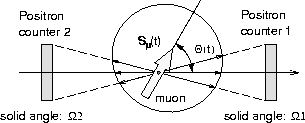We define the muon spin polarization along the counter axis as, where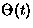is the time evolution of the muon spin angle relative to the counter axis (see Fig.8). The positron count rate of each counter Ni(t) (i=1,2) is found by integrating the positron angular distribution of this tilted muon spin over the counter solid angle and positron energy. The answer becomes:

where

Here,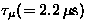is the muon lifetime,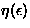is the detection efficiency of the positron counters and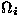is the solid angle of the counter i (=1,2). Ai is called the asymmetry of the individual counters, which is typically 0.2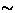0.3 in a conventional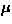SR setup.

The muon spin polarization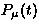, which is the information one would like to obtain from theSR technique, is calculated by taking the corrected asymmetry (eq.12), which is essentially the count difference of the two counters normalized by the total count: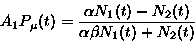(3)
where the parameter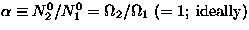corrects the deviation of the solid angles between the two counters, and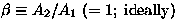corrects the difference of the counter asymmetries.

In Fig.9, an example of the positron counts [N1(t)] and the corrected asymmetry [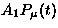] is shown. A Larmor precession of the muon spin is seen, as an external magnetic field perpendicular to the initial muon spin direction was applied during this measurement.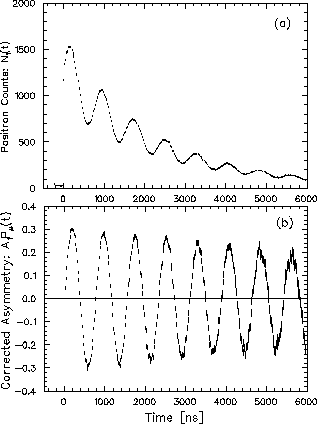The next section presents more details of the experimental setup which is required for theSR measurements.Next: 2.2 Experimental setup for Up: 2.1 The basics Previous: 2.1 The basics